# RS Aggarwal Solutions Class 6 Chapter 4 Integers Exercise 4B

In this chapter, we provide RS Aggarwal Solutions for Class 6 Chapter 4 Integers Exercise 4B for English medium students, Which will very helpful for every student in their exams. Students can download the latest RS Aggarwal Solutions for Class 6 Chapter 4 Integers Exercise 4B pdf, free RS Aggarwal Solutions Class 6 Chapter 4 Integers Exercise 4B book pdf download. Now you will get step by step solution to each question.

### RS Aggarwal Class 6 Chapter 4 Integers Ex 4B Download PDF

Question 1.
Solution:
(i) On the number line we start from 0 and move 9 steps to the right to reach a point A. Now, starting from A, we move 6 steps to the left to reach a point B, as shown below :Now, B represents the integer 3
9 + ( – 6) = 3
(ii) On the number line, we start from 0 and move 3 steps to the left to reach a point A. Now, starting from A, we move 7 steps to the right to reach a point B, as shown below :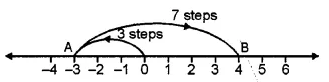And B represents the integer 4
( – 3) + 7 = 4
(iii) On the number line, we start from 0 and move 8 steps to the right to reach a point A. Now, starting from A, we move 8 steps to the left to reach a point B, as shown below :And, B represents the integer 0.
8 + ( – 8) = 0
(iv) On the number line, we start from 0 and move 1 step the left to reach a point A. Now, starting from point A, we move 3 steps to the left to reach g. point B, as shown below :And, B represents the integer – 4
( – 1) + ( – 3) = – 4.
(v) On the number line, we start from 0 and move 4 steps to the left to reach a point A. Now, starting from point A, we move 7 steps to the left to reach a point B, as shown below :And, B represents the integer -11.
( – 4) + ( – 7) = – 11
(vi) On the number line we start from 0 and move 2 steps to the left to reach a point A. Now, starting from A, we move 8 steps to the left to reach a point B, as shown below :And, B represents the integer – 10
( – 2) + ( – 8) = – 10
(vii) On the number line we start from 0 and move 3 steps to the right to reach a point A. Now, starting from A, we move 2 steps to the left to reach a point B and again starting from left to reach a point B and again starting from B, we move 4 steps to the left to reach a point C, as shown below :And, C represents the integer – 3
3 + ( – 2) + ( – 4) = – 3
(viii) On the number line we start from 0 and move 1 step to the left to reach a point A. Now, starting from A, we move 2 steps to the left to reach a point B and again starting from B, we move 3 steps to the left to reach point C, as shown below :And, C represents the integer – 6
( – 1) + ( – 2) + ( – 3) = – 6.
(ix) On the number line we start from 0 and move 5 steps to the right to reach a point A. Now, starting from A, we move 2 steps to the left to reach a point B and again starting from point B, we move 6 steps to the left to reach a point C, as shown below :And, C represents the integer – 3.
5 + (- 2) + (- 6) = – 3

Question 2.
Solution:
(i) (- 3) + ( – 9) = – 12
(Using the rule for addition of integers having like signs)
(ii) ( – 7) + ( – 8) = – 15
(Using the rule for addition of integers having like signs)
(iii) ( – 9) + 16 = 7
(Using the rule for addition of integers having unlike signs)
(iv) ( – 13) + 25 = 12
(Using the rule for addition of integers having unlike signs)
(v) 8 + ( – 17) = – 9
(Using the rule for addition of integers having unlike signs)
(vi) 2 + ( – 12) = – 10
(Using the rule for addition of integers having unlike signs)

Question 3.
Solution:
(i) Using the rule for addition of integers with like signs, we get: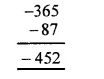(ii) Using the rule for addition of integers with like signs, we get :(iii) Using the rule for addition of integers with like signs, we get :(iv) Using the rule for addition of integers with like signs, we get:Question 4.
Solution:
(i) Using the rule for addition of integers with unlike signs, we get:(ii) Using the rule for addition of integers with unlike signs, we get:(iii) Using the rule for addition of integers with unlike signs, we have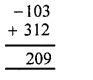(iv) Using the rule for addition of integers with unlike signs, we have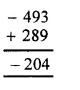Question 5.
Solution:
(i) Using the rule for addition of integers with unlike signs, we get :(ii) Using-the rule for addition of integers with unlike signs, we get(iii) Using the rule for addition of integers with unlike signs, we get :(iv) Using the rule for addition of integers with unlike signs, we get :(v) Using the rule for addition of integers with like signs, we get:(vi) Using the rule for addition of integers with unlike signs, we get :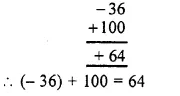(vii) Using the rule for addition of integers with unlike signs, we get :(viii) We have, ( – 18) + 25 + ( – 37)
= [( – 18) + 25] + ( – 37)
= 7 + ( – 37)
= – 30
(ix) We have, – 312 + 39 + 192
= ( – 312) + (39 + 192)
= ( – 312) + 231
= – 81
(x) We have ( – 51) + ( – 203) + 36 + ( – 28)
= [( – 51) + ( – 203)] + [36 + ( – 28)]
= ( – 254) + 8
= – 246

Question 6.
Solution:
(i) The additive inverse of – 57 is 57
(ii) The additive inverse of 183 is – 183
(iii) The additive inverse of 0 is 0
(iv) The additive inverse of – 1001 is 1001
(v) The additive inverse of 2054 is – 2054

Question 7.
Solution:
(i) Successor of 201 = 201 + 1 = 202
(ii) Successor of 70 = 70 + 1 = 71
(iii) Successor of – 5 = – 5 + 1 = – 4
(iv) Successor of – 99 = – 99 + 1 = – 98
(v) Successor of – 500 = – 500 + 1 = – 499 Ans.

Question 8.
Solution:
(i) Predecessor of 120 = 120 – 1 = 119
(ii) Predecessor of 79 = 79 – 1 = 78
(iii) Predecessor of – 8 = – 8 – 1 = – 9
(iv) Predecessor of – 141 = – 141 – 1 = – 142
(v) Predecessor of – 300 = – 300 – 1 = – 301 Ans.

Question 9.
Solution:
(i) ( – 7) + ( – 9) + 12 + ( – 16)
= – 7 – 9 + 12 – 16
= – 7 – 9 – 16 + 12
= – 32 + 12
= – 20
(ii) 37 + ( – 23) + ( – 65) + 9 + ( – 12)
= 37 – 23 – 65 + 9 – 12
= 37 + 9 – 23 – 65 – 12
= 46 – 100
= – 54
(iii) ( – 145) + 79 + ( – 265) + ( – 41) + 2
= – 145 + 79 – 265 – 41 + 2
= 79 + 2 – 145 – 265 – 41
= 81 – 451
= – 370
(iv) 1056 + ( – 798) + ( – 38) + 44 + ( – 1)
= 1056 – 798 – 38 + 44 – 1
= 1056 + 44 – 798 – 38 – 1
= 1100 – 837
= 263 Ans.

Question 10.
Solution:
Distance travelled from Patna to its north = 60 km
Distance travelled from that place to south of it = 90 km
Distance of the final place to Patna = 60 – 90
= – 30 km
= 30 km south
Ans.

Question 11.
Solution:
Total amount of pencils purchased = Rs. 30 + Rs. 25
= Rs 55
Total amount of pens purchased = Rs. 90
Total cost price = Rs. 55 + Rs. 90
= Rs. 145
Total sale price of pencils and pens = Rs 20 + Rs. 70
= Rs. 90
Loss = cost price – selling price
= Rs. 145 – Rs. 90
= Rs. 55 Ans.

Question 12.
Solution:
(i) True.
(ii) False : As if positive integer is greater then it will be positive.
(iii) True : As ( – a + a = 0).
(iv) False : As the sum of three integers can be zero or non-zero.
(v) False : As | – 5 | = 5 and | – 3 | = 3 and 5 ≮ 3.
(vi) False : | 8 – 5 | = | 3 | = 3 and | 8 | + | – 5 | = 8 + 5 = 13.

Question 13.
Solution:
(i) a + 6 = 0
Subtracting 6 from both sides,
a + 6 – 6 = 0 – 6
=> a = – 6
a = – 6.
(ii) 5 + a = 0
Subtracting 5 from both sides,
5 + a – 5 = 0 – 5
=> a = – 5
a = – 5
(iii) a + ( – 4) = 0
Adding 4 to both sides,
a + ( – 4) + 4 = 0 + 4
=> a = 4
a = 4
(iv) – 8 + a = 0
Adding 8 to both sides,
– 8 + a + 8 = 0 + 8
=> a – 8
a = 8 Ans.

All Chapter RS Aggarwal Solutions For Class 6 Maths

—————————————————————————–

All Subject NCERT Exemplar Problems Solutions For Class 6

All Subject NCERT Solutions For Class 6

*************************************************

I think you got complete solutions for this chapter. If You have any queries regarding this chapter, please comment on the below section our subject teacher will answer you. We tried our best to give complete solutions so you got good marks in your exam.

If these solutions have helped you, you can also share rsaggarwalsolutions.in to your friends.